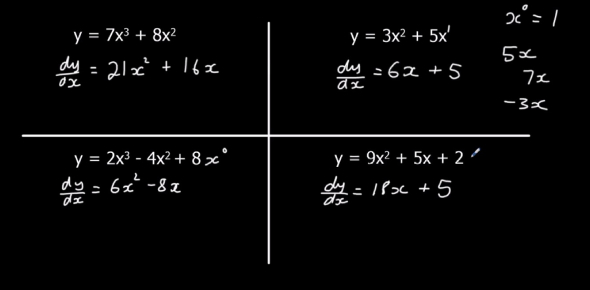# Differentiation Basic Rules Quiz

10 Questions | Attempts: 77SettingsDifferentiation is the derivative of a function of a real variable measuring the sensitivity to change of the function value. In this quiz, you will see how well do you understand the basic rules of differentiation and composite function. This quiz is specially designed to improve a person's mathematical skills. Go for it!

• 1.
Differentiate y= 4x-5
• A.

4

• B.

5

• C.

5

• D.

8

• 2.
Differentiate y= -x+4x2
• A.

-9x

• B.

-1+4x

• C.

-1+8x

• D.

8x

• 3.
Differentiate y =(2-4x)4
• A.

16(2-4x)4

• B.

-16(2-4x)3

• C.

4(2-4x)3

• D.

16(2-4x)3

• 4.
Differentiate y=2(4-x)5
• A.

10(4-x)4

• B.

52(4-x)

• C.

2(4-x)4

• D.

-10(4-x)4

• E.

Option 5

• 5.
Differentiate y= (12+3x)4
• A.

4(12+3x)

• B.

12(12+3x)3

• C.

(12+3x)3

• D.

4(12+3x)3

• 6.
Differentiate y=(2-x)6?
• A.

(2-x)5

• B.

6(2-x)

• C.

6(2-x)5

• D.

-6(2-x)5

• E.

Option 5

• 7.
Is differentiation of y=(3x-1)3 is 3(3x-1)2 true or false?
• A.

True

• B.

False

• 8.
Differentiate y=-5x3+4x2-x
• A.

15x2+8x-x

• B.

-15x2+8x-x

• C.

15x2+8x

• D.

-15x2+8x-1

## Related TopicsBack to top
×

Wait!
Here's an interesting quiz for you.